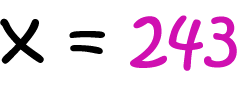# Definition of valueMathematics: a numeral assigned to a variable, or the result of a calculation.

The value of the variable $x$ on the left is $243$.

The value of $6 \div 2$ is $3$.Money: The amount something is worth.

For example, this coin has a value of $20$ cents.

### Description

The aim of this dictionary is to provide definitions to common mathematical terms. Students learn a new math skill every week at school, sometimes just before they start a new skill, if they want to look at what a specific term means, this is where this dictionary will become handy and a go-to guide for a student.

### Audience

Year 1 to Year 12 students

### Learning Objectives

Learn common math terms starting with letter V

Author: Subject Coach
You must be logged in as Student to ask a Question.